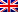# LP: canonical form and standard form

Contenus

## Canonical form and standard form

Linear programs follow certain rules when writing it. A linear program that follows the rules is said to be in canonical form. The simplex algorithm can only be applied to linear programs in canonical form.

## Canonic form

A linear program in its canonical form is:

• A Maximization problem, under Lower or equal constraints, all the variables of which are strictly positive.
• A problem of Minimization, under Greater or equal constraints, in which all the variables are strictly positive.

If the linear program does not correspond to these criteria, it is necessary to transform the constraints or the objective function according to the following operations:

• max z = - min -z
• x + y ≥ b is equivalent to - x - y ≤ - b
• x + y = b is equivalent to x + y ≥ b, x + y ≤ b

The canonical form is often represented in a matrix form:

• the vector of the coefficients of the objective function: c of size n
• the matrix of the coefficients of the left part of the constraints: A of size m * n
• the vector of the constants of the right part of the constraints: b of size m
• the vector of variables: x of size n

Thus the following linear program:

Written in the following form:

## Standard shape

For each inequality constraint of the canonical form, we add a positive deviation variable e such that:

Ax ≤ b ⇔ Ax + e = b, e ≥ 0, here e is a vector of size m of deviation variables.

Thus the canonical form is brought to the standard form by the addition of the variation variables in the vector of variables:

• the vector of the coefficients of the objective function: c of size n + m (n for x and m for e although the latter do not enter into the calculation)
• the matrix of the coefficients of the left part of the constraints: Ã of size m * (n + m), the right part of the matrix being an Identity matrix of size m.
• the vector of the constants of the right part of the constraints: b of size m
• the vector of variables of size n + m

Some inequalities do not make it possible to have positive base variables. For this we must add other variables called excess and artificial variables.

Here are the rules to follow to best transform the simplex into standard form:

• constraints "greater than or equal" or "less than or equal with b negative": make b positive and add "- deviation variable + artificial variable"
• "equality" constraints: add "+ artificial variable"
• constraints “less than or equal with positive b”: add “+ variation variable” as seen previously.FRFRENRUES